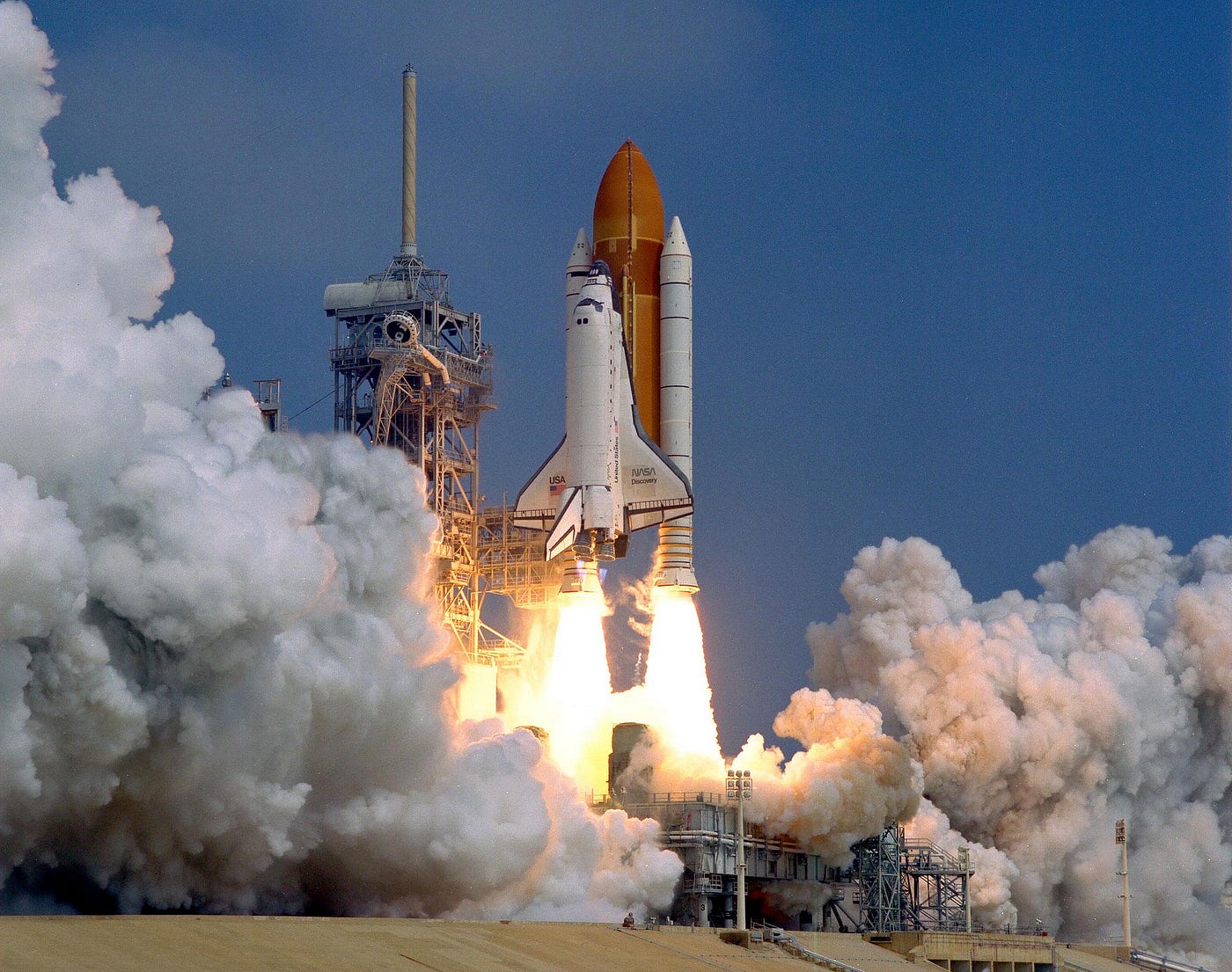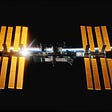# Rocket Science 101: The tyranny of the rocket equation

## How the giant leap for mankind is not the first step on the Moon but attaining Earth orbit

The Universe is governed by the laws of physics that cannot be changed by us. As such, there are hard limits to what we can do with rockets and how we build them. The working of rockets is governed by the Tsiolkovsky rocket equation, named after the rocket scientist Konstantin Tsiolkovsky. This article is supposed to act as a basic introduction to variables governing rocket science and their implications. As such, some generalizations will be made.

# Knowing the players

Before we get to the rocket equation, let’s have a look at the governing players. There are two primary things affecting a rocket’s journey to outer space: `Delta-v` and `Exhaust velocity`.

## Delta-v

To reach outer space, rockets need to spend energy against Earth’s (or another object’s) gravity. This energy is often expressed as `delta-v`.

The `delta-v` generally depends on how far away from Earth you want to go (Low Earth Orbit, the Moon, Mars, etc.). It also increases the deeper you want to go in a gravitational well (says Earth’s surface to the Moon’s surface). The `delta-v` will thus define the energy needed to reach the destination.

The approximate `delta-v` required to reach different destinations in the solar system (calculated using vis-viva equation) is as follows:

`1. Earth’s surface to Low Earth Orbit (LEO) = 9.3 km/s (at 250 km)2. LEO to Low Lunar Orbit (LLO) = 4 km/s3. LEO to surface of the Moon = 5.9 km/s4. LEO to Mars Transfer Orbit = 4 km/s5. LEO to the surface of Mars = 10.4 km/s`

A few interesting things come up here:

• It takes more than double the `delta-v` to reach Low Earth Orbit (LEO) from Earth’s surface than it takes to reach Low Lunar Orbit (LLO) from LEO.
• All destinations between the LEO and the Moon are but a fraction of that required to just reach LEO from the Earth’s surface.
• Earth’s surface to LEO is also nearly equal to that required from LEO to surface of Mars.

This is remarkable as it means that the first barrier to space (Earth’s surface to LEO) is much higher than the subsequent ones. It is so high because of the magnitude of Earth’s gravity.

The giant leap for mankind was thus not stepping on the Moon, but attaining Earth orbit!

## Exhaust velocity

The energy available from the type of propulsion system is often expressed as `Exhaust velocity`. This is used to achieve the `delta-v` required for a mission.

Rocket propulsion systems come in a wide variety. Most rocket engines use chemical propellants. Chemical elements which react energetically (under various conditions) are the ones chosen for propulsion as they give high exhaust velocities. Different combinations of propellants give different exhaust velocities due to different energy efficiencies.

Both `delta-v` and `Exhaust velocity` are expressed in the same units (km/s) for easier comparison. Here are major chemical propulsion systems currently in use and their average exhaust velocities.

`1. Solid propellant = 3 km/s(E.g. The Space Shuttle)2. Kerosene-Oxygen = 3.1 km/s(E.g. Falcon, Soyuz, Long March 6, Saturn V)3. Hypergols (propellants that ignite on contact) = 3.2 km/s(E.g. PSLV, Proton)4. Hydrogen-Oxygen = 3.4 km/s(E.g. Ariane 5, Delta IV)`The Space Shuttle boosters (at the sides) used solid propellant. Source: NASA Flickr

`Specific impulse` defines how effectively a rocket uses propellant. A propulsion system with a higher specific impulse is more efficient and therefore less propellant mass is needed for a given `delta-v`.

`Note: Higher specific impulse or exhaust velocity alone isn't enough to get something out of an object's gravitational well. The amount of thrust generated by the engine should be high too, which is why the low-thrust ion engines (despite having high specific impulse) can't get rockets out of Earth's gravity.`

And `specific impulse` is simply the `exhaust velocity` relative to the rocket. So a rocket is generally more efficient if it has better exhaust velocities, assuming the total mass of the rocket is the same. However, different types of propellants bring with them different structural requirements which may increase the mass. This leads to the third factor called `Mass ratio`.

## Mass ratio

`Mass ratio` is the total rocket mass for a given destination divided by the dry rocket mass (i.e without the propellant). Higher mass ratios mean the amount of propellant required is enormously more than the rest of the rocket. This brings us to what is famously known as the rocket equation which constraints how much payload the rocket can carry to a given destination.

# The Rocket Equation

The rocket equation relates the three quantities discussed above as:

`mass ratio = e ^ (delta-v/exhaust velocity),where 'e' is the mathematical constant equal to ~2.72`

There are intricate consequences of the rocket equation which might not be obvious at first glance. The mass ratio directly depends on the `delta-v` and `exhaust velocity`. Have a look at the graph below derived from the rocket equation. It compares `(delta-v/exhaust velocity)` to the `mass ratio`.

For a given destination, there are two scenarios:

`1. If delta-v <= exhaust velocity, the mass ratio is low and large payloads are thus possible.2. If delta-v > exhaust velocity, the mass ratio exponentially increases and only tiny payloads are allowed. Most of the ship will be propellant mass.`

The `mass ratio` can thus spiral out of control very quickly. As the graph above shows, for a `(delta-v/exhaust velocity)` value of 3, the required mass ratio is a whopping 20! That means the rocket will carry 20 times more fuel than the rest of the mass of the rocket! Slowly it gets harder and harder to get out of the Earth’s gravitational influence.

Around this area, we end up with rockets having more than 80–90% as just the propellant. Even the mighty Saturn V that put astronauts on the Moon was 85% propellant and 15% rocket. An even lesser percentage is the payload mass which is similarly related.

Basically, throwing things into space is really expensive and inefficient.

# The Tyranny of the Rocket Equation

If the radius of the Earth were larger (~ 9700 km), the `delta-v` requirement would be very high and the mass fraction would be enormous. Due to practical limits of engineering, even the most energetic chemical propellant (hydrogen-oxygen) would not be able to make a rocket reach space. There would be no space program of the type we have now i.e. which uses chemical propellants. The only way to solve this problem would be to go beyond chemical propulsion (E.g. Nuclear propulsion). Good thing that Earth is not big enough I guess!

If the Earth was 50% larger, there would be no space program of the type we have now.

# To the Moon

However, even for us, there are implications of the limitations on the way rockets work. Because the Earth’s gravitational pull is still large enough that our chemical propellant rockets can never be a lot more efficient, the Moon becomes an interesting place.

Being able to extract raw materials of the Moon and harness them would free us from the need to drag everything to space from Earth’s large gravitational pull. The Moon has a much lower `delta-v` requirement to go to various destinations in the solar system, thereby putting the consequences of the rocket equation in our favor. We have an article on the same linked below.

--

--

--

## More from TeamIndus Blog

Privately funded mission to land on the Moon in 2020

## How Can We Create an Atmosphere on Mars?## Summer Starts and the Summer of Mars Starts, too, View Bright Vesta, and Venus Avoids the Beehive!## The Waxing Moon Slides by Some Stars, Bright Planets Parade all night, and Asterisms Abound!## The Panic Caused When A Space Lab Crashed Into The Earth## Saeko Hayashi: a Japanese astronomer in Hawaii## Why We Can Expect 5 Years of Stormy Solar Weather## Satellite Defense Contracts Awarded to SpaceX and York Space Systems## How Zeno’s Paradox was resolved: by physics, not math alone## How the Sun Will Die. And Why.## What Would a Sustainable Society Look Like?## We’ve Finally Seen the Black Hole at the Centre of the Milky Way